# Absolute References in Excel - A Beginner's GuideAgnes Lo

## What is an absolute reference?

Absolute reference Excel definition: An absolute reference in Excel means there is a fixed point of reference applied to a cell or a formula. This is so the return value will always stay the same no matter where the cell or the formula moves to — within the same sheet or across different sheets.

Use this free Excel file to practice absolute references along with the tutorial.

For example, in the image below, all employees will receive the same bonus payout amount of \$1500. The amount of \$1500 is the constant in this situation, and an absolute reference can be used to help calculate the total payout of salary (a figure that is different for each person) plus bonus payout.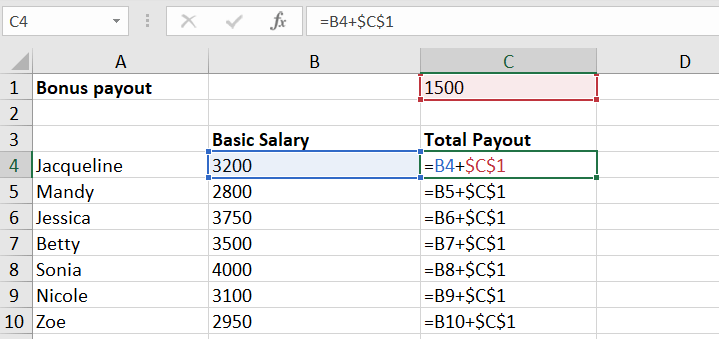In Excel, all references are relative by default. To apply absolute reference in Excel, the addition of a dollar sign (\$) is required in the Excel formula — i.e., =\$C\$1 as shown in the example above.

Without the dollar sign (\$) in the formula, Excel naturally interprets the cell address as a relative reference, where the point of reference changes as the relative row and column coordinates move.

The idea of an absolute cell reference is to hold a specific cell constant, so the value remains the same when being copied to other cells. The absolute reference Excel feature is an indispensable tool to save you time and effort when working with spreadsheets.

## A quick recap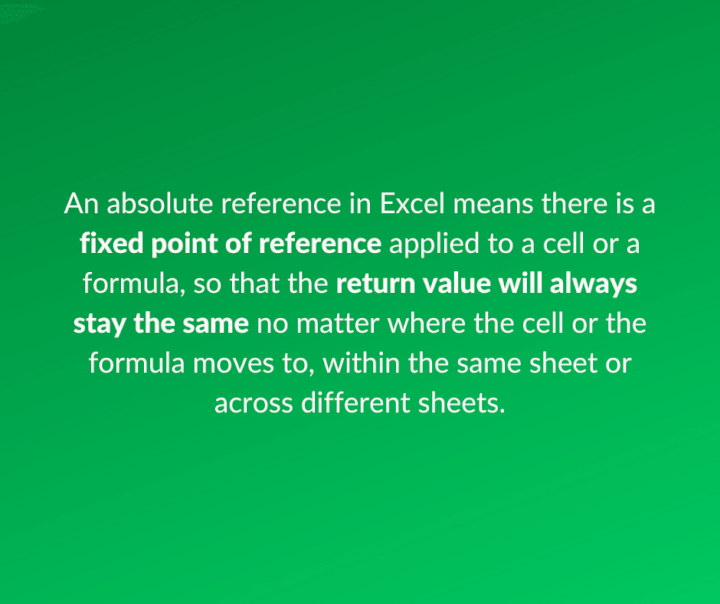## How to make an absolute reference in Excel

Here are the steps on how to make a basic absolute reference in Excel - \$A\$1:

1. Choose a cell where you would like to create an absolute reference. Cell A1 in this example: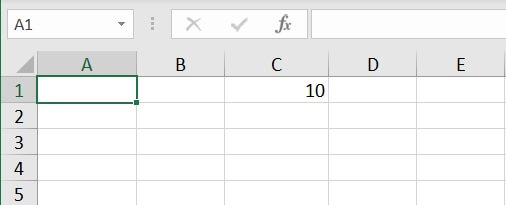1. In the formula of Cell A1, Enter “=” (the equal sign) and then select the point of reference - Cell C1.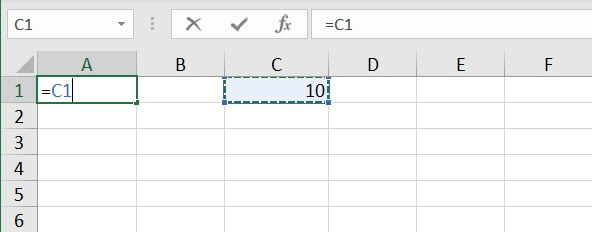1. In the same formula, either manually add two dollar signs (SHIFT + 4) in front of the row and column coordinates, or press the F4 key as a shortcut.
2. Press ENTER and now Cell A1 has the value of Cell C1 as a fixed point of reference.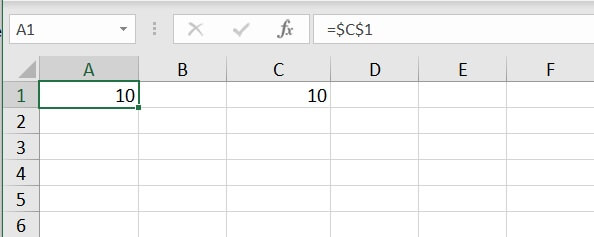## Examples of absolute reference

Because of its versatile nature, absolute reference is one of the most frequently used functions in Excel. It can be used with both numbers and text.

Absolute numbers are very popular in calculations in Excel, as it keeps a cell constant so that users can make use of the point of reference in various scenarios and forecasts.

Let’s take a look at the example below:

A US-based stationery company has found a new buyer based in Germany. They need to work out a proposal for the new partner. Given that there are differences in terms of currencies, they would like to include the unit cost in EUR for a complete picture of the costs.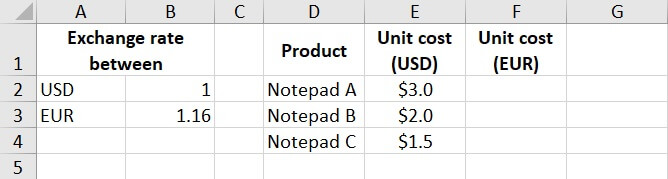This means all of those three unit costs in USD have to be multiplied by the exchange rate of EUR-USD — unit cost (USD) * EUR-USD exchange rate.

There are three unit costs, and one exchange rate. The exchange rate of EUR (Cell B3) has to be held constant when applying the formulas under column F for the unit costs in EUR.

To begin with, find out the unit cost of Notepad A in EUR:

1. Enter: “=E2*B3”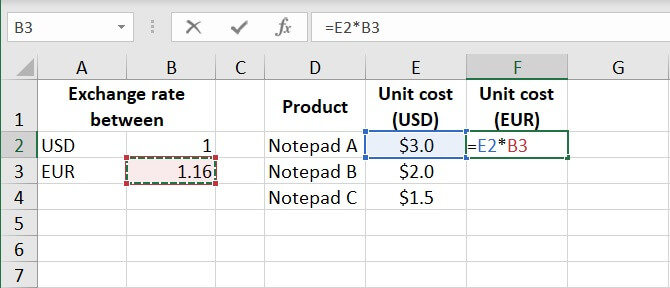1. Manually insert the \$ in front of both row and column coordinates or press F4 to create an absolute reference, then press ENTER.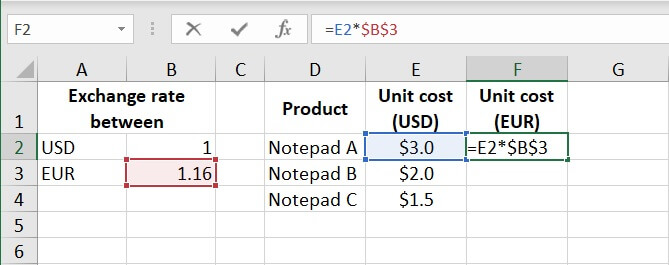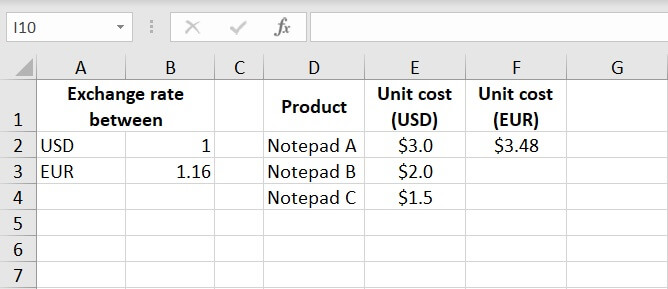1. To find out other unit costs in EUR, drag down Cell F2 to apply the formulas to F3 and F4 as well.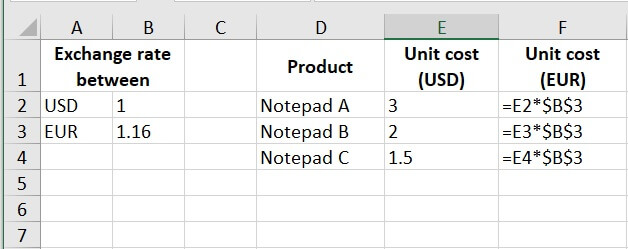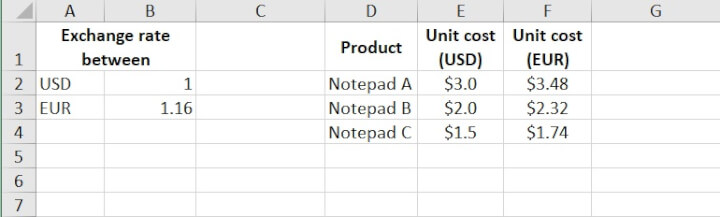With the use of absolute reference, the value of B3 becomes a constant for all unit costs.

It provides a quick solution to the scenario on hand and minimizes mistakes by doing the multiplication by hand manually for each cell.

If there was no absolute reference in the formulas, Excel would return \$0 as the unit cost as we drag down Cell F2. Because the reference point would become relative and change across cells, and there are no other values in Cell B4 and B5.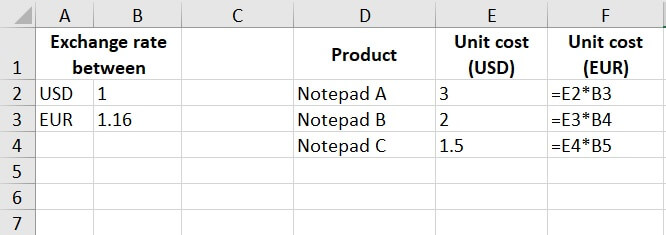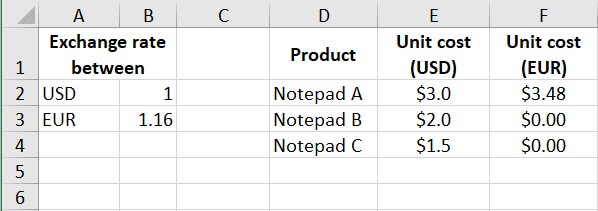## Absolute reference vs relative reference in Excel

Let's compare the differences between absolute and relative references, with examples of when to use each.

### Absolute reference Excel

This refers to a fixed point of reference, is a constant, and involves the use of dollar sign \$ in the formula (i.e., everyone is to receive the same bonus payout, so the amount \$1500 is the constant in this situation).### Relative reference Excel

This refers to a relative point of reference, is constantly changing, and dollar sign \$ is absent in the formula (i.e., when each unit price and qty are difference variables, there’s no constant in the calculation).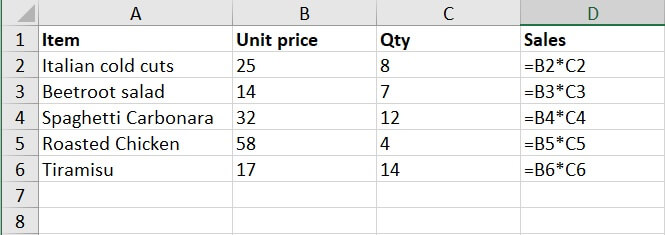## A quick hint

Press the F4 key as a shortcut to make an absolute cell reference in Excel.

## Summary

Absolute reference is applied in situations where the same value is used throughout different scenarios or variables. The point of reference is a constant that remains the same.

The dollar sign \$ is employed to create an absolute reference. Without it, it is a relative reference, no cell is ‘locked’.

It is commonly applied in a mix of formulas and functions in Excel, and works with both numbers and text. It’s particularly useful when doing complicated calculations and formulas. It helps minimize errors and saves time to input formulas by hand, one by one.

To learn more about absolute references and other essential Excel features try our Basic and Advanced Excel course today. Or start learning some Excel basics with our free Excel in an Hour course.